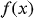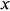1
108
views
4
Problem

Problem 4

Chapter 1: Functions and ModelsTextbook ExpertVerified Tutor
13 Oct 2021

Given information

The functionis given by the following table:1 2 3 4 5 61 1.9 2.8 3.5 3.1 2.9

Step-by-step explanation

Step 1.

Here, the set of all input values for the given function is 1, 2, 3, 4, 5, 6.

From the given table, we can observe that the different input values have different output values.

That is, no two different preimages have same image.

Hence the given function is one-to-one.